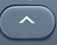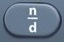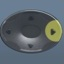# Cubing3

Number Cubed = Number × Itself × Itself = Number³

Basics

Number Cubed = Number × Itself × Itself = Number³

5 cubed = 5 × 5 × 5 = 5³ = 125
12 cubed = 12 x 12 x 12 = 12³ = 1728
20 cubed = 20 x 20 x 20 = 20³ = 80003   is the cubing command.

Formulas (NOT on Mathematics Formula Sheet)
Number Cubed = Number × Itself × Itself = Number³

Question

What is 4³?

64

Input Display Commentblinker clears screen3 4 cubed64 Answer
###### Number³

Question

What is$\bf\displaystyle\frac{1}{4}$ cubed?$\bf\displaystyle\frac{1}{64}$

Input Display Commentblinker clears screen143$\bf\displaystyle{{\frac{1}{4}}^{3}}$$\bf\displaystyle\frac{1}{4}$ cubed$\bf\displaystyle\frac{1}{64}$ Answer
###### Number³

Question

What is 71.4 cubed?

363994.334

Input Display Commentblinker clears screen
71.43 71.4³ 71.4 cubed363994.344 Answer
###### Number³

Practice – Questions
1.  What is 7³?

2.  What is 13³?

3.  What is$\bf\displaystyle\frac{1}{2}$ cubed?

4.  What is$\bf\displaystyle\frac{3}{4}$ cubed?

5.  What is 313.25 cubed?

3.$\bf\displaystyle\frac{1}{8}$
4.$\bf\displaystyle\frac{27}{64}$